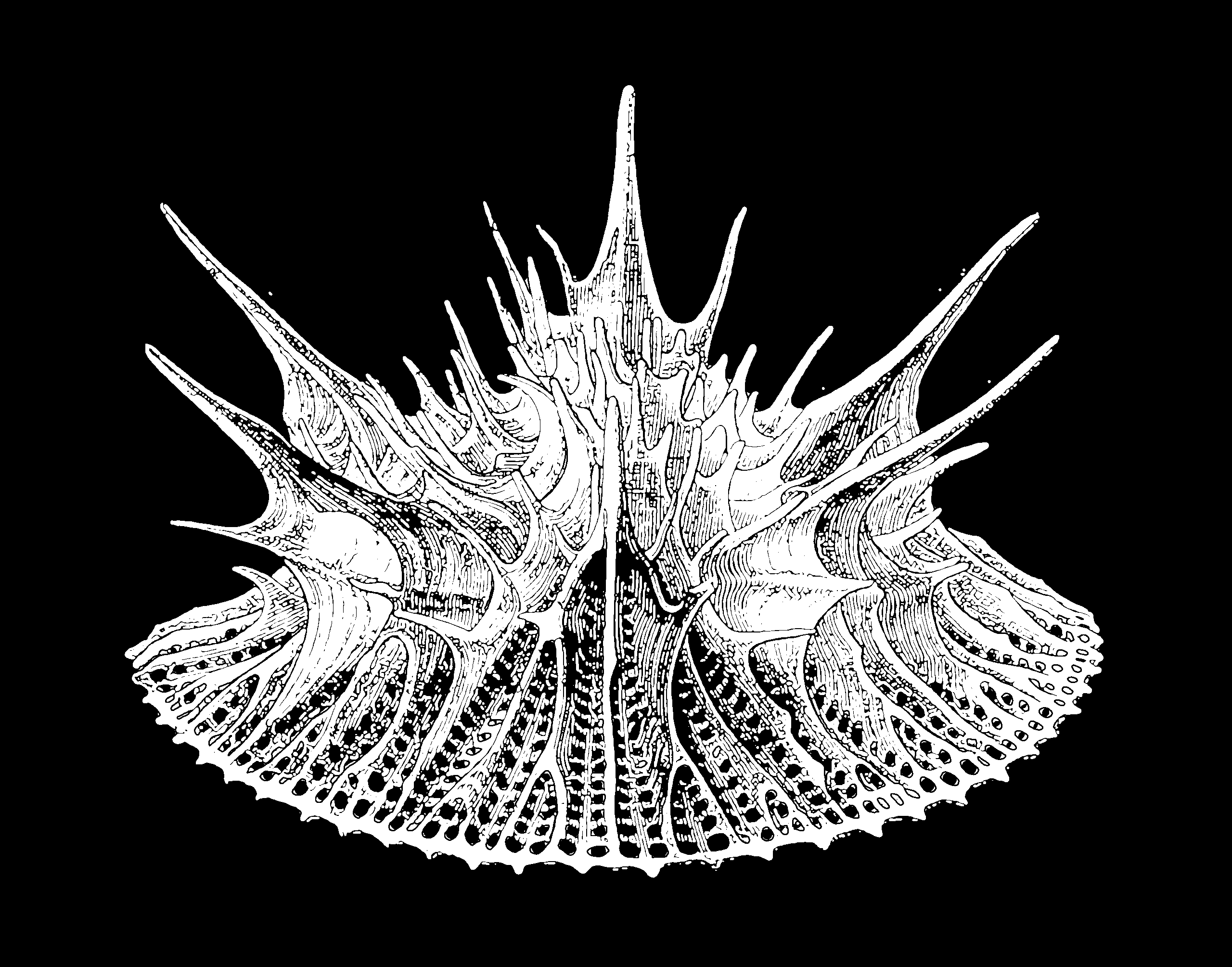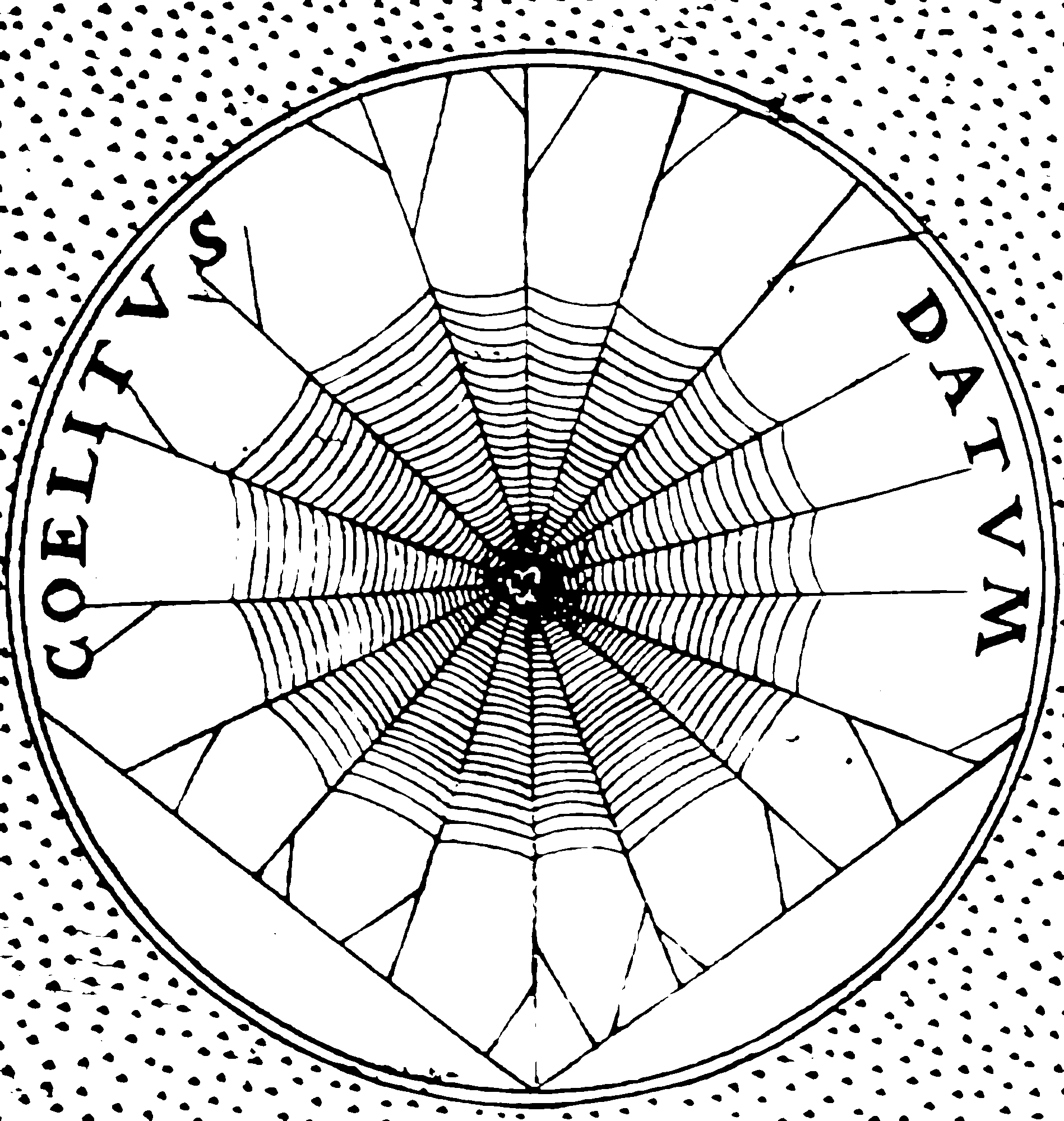# Multivariate Gamma distributions

$\renewcommand{\var}{\operatorname{Var}} \renewcommand{\corr}{\operatorname{Corr}} \renewcommand{\dd}{\mathrm{d}} \renewcommand{\bb}{\mathbb{#1}} \renewcommand{\vv}{\boldsymbol{#1}} \renewcommand{\rv}{\mathsf{#1}} \renewcommand{\vrv}{\vv{\rv{#1}}} \renewcommand{\disteq}{\stackrel{d}{=}} \renewcommand{\gvn}{\mid} \renewcommand{\mm}{\mathrm{#1}} \renewcommand{\Ex}{\mathbb{E}} \renewcommand{\Pr}{\mathbb{P}}$

Various multivariate distributions that are marginally Gamma distributed but have correlations. No unifying insight here, this is a grab-bag right now.

How general can the joint distribution of a Gamma vector be? Much effort has been spent on this question and several more below. There are lots of slightly different options, is the short version.So here is the simplest multivariate case:

## Bivariate thinned Gamma RVs

There are many possible ways of generating two correlated Gamma variates, but as far as I am concerned the default is the Beta thinning method.

For this and throughout, we use the fact that \begin{aligned} \rv{g}&\sim\operatorname{Gamma}(a,\lambda)\\ &\Rightarrow\\ \Ex[\rv{g}]&=\frac{a}{\lambda}\\ \var[\rv{g}]&=\frac{a}{\lambda^2}\\ \Ex[\rv{g}^2]&=\frac{a^2 +a}{\lambda^2}. \end{aligned}

Suppose we have $$\rv{g}_1\sim\operatorname{Gamma}(a_1+a_2,\lambda)$$ and $$\rv{b}\sim\operatorname{Beta}(a_1, a_2)$$ and $$\rv{g}'\sim\operatorname{Gamma}(a_2,\lambda)$$ with all rvs jointly independent. Now we generate $\rv{g}_2=\rv{b}\rv{g}_1+\rv{g}'.$ Then \begin{aligned}\Ex[\rv{g}_2] &=\Ex[\rv{b}\rv{g}_1]+\Ex[\rv{g}']\\ &=\frac{a_1+a_2}{\lambda} \end{aligned} and \begin{aligned}\Ex[\rv{g}_1\rv{g}_2] &=\Ex[\rv{g}_1(\rv{b}\rv{g}_1+\rv{g}')]\\ &=\Ex[\rv{g}_1\rv{b}\rv{g}_1+\rv{g}_1\rv{g}')]\\ &=\Ex[\rv{g}_1^2\rv{b}]+\Ex[\rv{g}_1\rv{g}')]\\ &=\Ex[\rv{g}_1^2]\Ex[\rv{b}]+\Ex[\rv{g}_1]\Ex[\rv{g}')]\\ &=\frac{(a_1+a_2)^2 + (a_1+a_2)}{\lambda^2}\frac{a_1}{a_1+a_2}+\frac{a_1+a_2}{\lambda}\frac{a_2}{\lambda}\\ &=\frac{a_1(a_1+a_2) + a_1}{\lambda^2}+\frac{a_2(a_1+a_2)}{\lambda^2}\\ &=\frac{a_1(a_1+a_2) + a_1+ a_2(a_1+a_2)}{\lambda^2}\\ &=\frac{a_1^2+2a_1a_2 + a_1+a_2^2}{\lambda^2} \end{aligned} and thus \begin{aligned}\var[\rv{g}_1,\rv{g}_2] &=\Ex[\rv{g}_1\rv{g}_2]-\Ex[\rv{g}_1]\Ex[\rv{g}_2]\\ &=\frac{a_1^2+2a_1a_2 + a_1+a_2^2-(a_1+a_2)^2}{\lambda^2}\\ &=\frac{a_1^2+2a_1a_2 + a_1+a_2^2-a_1^2-2a_1a_2-a_2^2}{\lambda^2}\\ &=\frac{a_1}{\lambda^2} \end{aligned} Thence \begin{aligned}\corr[\rv{g}_1,\rv{g}_2] &=\frac{\var[\rv{g}_1,\rv{g}_2]}{\sqrt{\var[\rv{g}_1]\var[\rv{g}_2]}}\\ &=\frac{\frac{a_1}{\lambda^2}}{\frac{a_1+a_2}{\lambda^2}}\\ &=\frac{a_1 }{a_1+a_2}. \end{aligned}

We can use this to generate more than two correlated gamma variates by successively thinning them, as long as we take care to remember that we can only add independent Gamma variates together to produce a new Gamma variate in general (unlike, say, Gaussian processes.)

## Multivariate latent thinned Gamma RVs

A different construction.

Suppose we have $$K$$ independent latent Gamma variates. $\rv{\zeta}_k \sim \operatorname{Gamma}(A, \lambda), k=1,2,\dots,K.$ Let us also suppose we have a $$\mathbb{R}^{D\times K}$$ latent mixing matrix, $$\mm{R}=[r_{d,k}]$$ whose entries are all non-negative and whose rows sum to $$A.$$ Now, let us define new random variates $\rv{g}_d =\sum_{k=1}^K \rv{b}_{d,k}\rv{\zeta}_k, d=1,2,\dots,D.$ where $$\rv{b}_{d,k}\sim\operatorname{Beta}(r_{d,k},A-r_{d,k}).$$ By construction and the Beta thinning rule, we know that $\rv{g}_d \sim \operatorname{Gamma}(A, \lambda), d=1,2,\dots,D.$ What can we say about the correlation between them?

For this, we need the fact that \begin{aligned} \rv{b}&\sim\operatorname{Beta}(\alpha,\beta)\\ &\Rightarrow\\ \Ex[\rv{b}]&=\frac{\alpha}{\alpha+\beta}\\ \var[\rv{b}]&=\frac{\alpha\beta}{(\alpha+\beta)^2(\alpha+\beta+1)}\\ \Ex[\rv{b}^2]&=\frac{\alpha^2(\alpha+\beta+1)+\alpha\beta}{(\alpha+\beta)^2(\alpha+\beta+1)} \end{aligned}

Expanding out the $$\rv{g}$$s, \begin{aligned}\Ex[\rv{g}_1\rv{g}_2] &=\Ex\left[\left(\sum_{k=1}^K \rv{b}_{1,k}\rv{\zeta}_k\right)\left( \sum_{k=1}^K \rv{b}_{2,k}\rv{\zeta}_k\right)\right]\\ &=\Ex\left[\sum_{k=1}^K \sum_{j=1}^K \rv{b}_{1,k}\rv{\zeta}_k\rv{b}_{2,j}\rv{\zeta}_j\right]\\ &=\sum_{k=1}^K \sum_{j=1}^K \Ex\left[\rv{b}_{1,k}\rv{\zeta}_k\rv{b}_{2,j}\rv{\zeta}_j\right]\\ &=\sum_{k=1}^K \Ex\left[\rv{b}_{1,k}\rv{b}_{2,k}\rv{\zeta}_k^2\right] + 2\sum_{k=1}^K \sum_{j=k+1}^K \Ex\left[\rv{b}_{1,k}\rv{\zeta}_k\rv{b}_{2,j}\rv{\zeta}_j\right]\\ &=\sum_{k=1}^K \Ex[\rv{b}_{1,k}]\Ex[\rv{b}_{2,k}]\Ex[\rv{\zeta}_k^2] + 2\sum_{k=1}^K \sum_{j=k+1}^K \Ex[\rv{b}_{1,k}]\Ex[\rv{\zeta}_k]\Ex[\rv{b}_{2,j}]\Ex[\rv{\zeta}_j]\\ &=\frac{A^2+A}{\lambda^2 A^2}\sum_{k=1}^Kr_{1,k}r_{2,k} + 2\sum_{k=1}^K \sum_{j=k+1}^K \frac{r_{1,k}}{A} \frac{A}{\lambda} \frac{r_{2,j}}{A} \frac{A}{\lambda} \\ &=\frac{A+1}{\lambda^2 A}\sum_{k=1}^Kr_{1,k}r_{2,k} + 2\sum_{k=1}^K \sum_{j=k+1}^K \frac{r_{1,k}r_{2,j}}{\lambda^2} \\ &=\frac{1}{\lambda^2}\left(\frac{A+1}{ A}\sum_{k=1}^Kr_{1,k}r_{2,k} + 2\sum_{k=1}^K \sum_{j=k+1}^K r_{1,k}r_{2,j} \right)\\ &=\frac{1}{\lambda^2}\left(\frac{1}{ A}\sum_{k=1}^Kr_{1,k}r_{2,k} + \sum_{k=1}^K \sum_{j=1}^K r_{1,k}r_{2,j} \right)\\ &=\frac{\frac1A \vv{r}_1\cdot\vv{r}_2+A^2}{\lambda^2} \end{aligned}

So \begin{aligned}\var[\rv{g}_1,\rv{g}_2] &=\Ex[\rv{g}_1\rv{g}_2]-\Ex\rv{g}_1\Ex\rv{g}_2\\ &=\frac{ \vv{r}_1\cdot\vv{r}_2}{A\lambda^2}. \end{aligned} and \begin{aligned}\corr[\rv{g}_1,\rv{g}_2] &=\frac{\var[\rv{g}_1,\rv{g}_2]}{\sqrt{\var[\rv{g}_1]\var[\rv{g}_2]}}\\ &=\frac{\frac{ \vv{r}_1\cdot\vv{r}_2}{A\lambda^2}}{\frac{A}{\lambda^2}}\\ &=\frac{ \vv{r}_1\cdot\vv{r}_2}{A^2}. \end{aligned}

After all that work, we find pretty much what we would have expected for the correlation of rvs constructed by weighting of latent Gaussian factors. Was there a shortcut that could have gotten us there quicker?

## Kingman constructionsKingman (1967), Lijoi and Prünster (2010). TBD. See

### Random correlated weights

Ranganath and Blei (2018) creates random measures that seems to induce Gamma weights by imposing a Gaussian correlation structure on the random weights of a random selection of Poisson atoms. This seems to still be a Kingman construction?

## Generalized Multivariate Gamma

Das and Dey (2010) discusses a lineage of Generalized Multivariate Gammas that sound interesting. For them, multivariate appears to mean square-matrix-variate, but in the papers they cite, it seems to be vector-valued. What is going on here? Am I being boneheaded? Lukacs and Laha (1964) is also referenced; what does that say?

## Dussauchoy Multivariate GammaDefined in terms of Characteristic Function, to have analogous joint independence properties to the multivariate Gaussian .

## Gaver Multivariate Gamma

Some kind of negative-binomial mixture?

## Maximally general multivariate Gamma

The paper to rule them all is Pérez-Abreu and Stelzer (2014), wherein we get a (maximally?) general $$d$$-variate Gamma distribution in terms of two functions $$\alpha$$ and $$\lambda$$ on the unit sphere $$\mathbf{S}_{\mathbb{R}^{d},\|\cdot\|}$$ with respect to the norm $$\|\cdot\|$$. In fact $$\alpha$$ is a Borel measure, so we more properly say it is a function on the Borel subsets of the unit sphere, and $$\lambda$$ must be Borel-measurable. Enough of that; I play loose with measure-theoretic niceties from here on.

Now, let’s match the notation of the original paper. We use the convention that the Fourier transform $$\widehat{\mu}$$ of a measure $$\mu$$ on $$\mathbb{M}=\mathbb{R}^{d}$$ is given in terms of inner product $$(\omega, x)\equiv x^\top\omega$$. $\hat{\mu}(\omega)=\int_{\mathbb{M}} e^{i(\omega, x)} \mu(\mathrm{d} x) \quad \omega \in \mathbb{M}.$ The following theorem then characterizes multivariate Gamma distributions in terms of these Fourier transforms.

Let $$\mu$$ be an infinitely divisible probability distribution on $$\mathbb{R}^{d} .$$ If there exists a finite measure $$\alpha$$ on the unit sphere $$\mathbf{S}_{\mathbb{R}^{d},\|\cdot\|}$$ … and a Borel-measurable function $$\lambda: \mathbf{S}_{\mathbb{R}^{d},\|\cdot\|} \rightarrow \mathbb{R}_{+}$$ such that the Fourier transform of the [probability] measure is given $\hat{\mu}(\omega)=\exp \left(\int_{\mathbf{S}_{\mathbb{R}^{d},\|\cdot\|}} \int_{\mathbb{R}_{+}}\left(e^{i r v^{\top} \omega}-1\right) \frac{e^{-\lambda(v) r}}{r} \mathrm{~d} r \alpha(\mathrm{d} v)\right)$ for all $$\omega \in \mathbb{R}^{d}$$, then $$\mu$$ is called a $$d$$-dimensional Gamma distribution with parameters $$\alpha$$ and $$\lambda$$, abbreviated $$\Gamma_{d}(\alpha, \lambda)$$ distribution. If $$\lambda$$ is constant, we call $$\mu$$ a $$\|\cdot\|$$-homogeneous $$\Gamma_{d}(\alpha, \lambda)$$-distribution.

Intriguingly the choice of which norm $$\|\cdot\|$$ doesn’t seem to matter much; changing the norm just perturbs $$\lambda$$ in a simple fashion. I assume that $$L_2$$ is easiest in practice; integral of polynomials on those are straightforward at least.

It is not clear to me what families of $$\lambda$$s and $$\alpha$$s are interesting from that description. If we could get that inner integral $$\int_{\mathbb{R}_{+}}\left(e^{i r v^{\top} \omega}-1\right) \frac{e^{-\lambda(v) r}}{r} \mathrm{~d} r$$ to look polynomial we might do OK.

We do know which ones are admissible, at least:

Let $$\alpha$$ be a finite measure on $$\mathbf{S}_{\mathbb{R}^{d},\|\cdot\|}$$ and $$\lambda: \mathbf{S}_{\mathbb{R}^{d},\|\cdot\|} \rightarrow \mathbb{R}_{+}$$ a measurable function. Then [the previous construction] defines a Lévy measure $$v_{\mu}$$ and thus there exists a $$\Gamma_{d}(\alpha, \lambda)$$ probability distribution $$\mu$$ if and only if $\int_{\mathbf{S}_{\mathbb{R}}^{d},\|\cdot\|} \ln \left(1+\frac{1}{\lambda(v)}\right) \alpha(\mathrm{d} v)<\infty .$ Moreover, $$\int_{\mathbb{R}^{d}}(\|x\| \wedge 1) v_{\mu}(\mathrm{d} x)<\infty$$ holds true. The condition is trivially satisfied, if $$\lambda$$ is bounded away from zero $$\alpha$$-almost everywhere.

Le us fix $$\|v\|=\sqrt{v^\top v}$$ and consider that homogenous case where $$\lambda$$ is constant on the $$\|v\|=1$$ sphere? Then the term with $$\lambda$$ simplifies to a weird complex logarithm on that same sphere \begin{aligned} \hat{\mu}(\omega) &=\exp \left(\int_{\mathbf{S}_{\mathbb{R}^{d},\|\cdot\|}} \int_{\mathbb{R}_{+}}\left(e^{i r v^{\top} \omega}-1\right) \frac{e^{-r \lambda }}{r} \mathrm{~d} r \alpha(\mathrm{d} v)\right)\\ &=\exp \left(\int_{\mathbf{S}_{\mathbb{R}^{d},\|\cdot\|}} \left(\ln \frac{\lambda}{\lambda - i v^\top\omega} \right) \alpha(\mathrm{d} v)\right) \end{aligned}

This is a weird creature. Note that everything cancels out if $$\omega=0$$. How flexible is that? If we fix some $$d$$, we can get a $$d$$-point gamma correlation by choosing different measures on the sphere in $$\mathbb{R}^d$$.

### No comments yet. Why not leave one?

GitHub-flavored Markdown & a sane subset of HTML is supported.